×

Search anything:

# Bag of Words (BoW) in NLP

#### Natural Language Processing (NLP)Get this book -> Problems on Array: For Interviews and Competitive Programming

## Intoduction

In this article, the concept of data preprocessing had been touched upon with a special emphasis on Stop Words. Furthermore, the concept of Bag of Words was used as well in a supporting role. This article shall serve as a detailed guide on the same for anyone who wishes to form an understanding of the same.

The Bag of Words technique falls under the category of text representation in NLP, wherein the words are converted to numerical values which can be understood and used by algorithms. This is a necessary step as human languages can't be directly fed to algorithms which work on numerical data.

Moving ahead, the Bag of Words method is a well-known NLP tool for transforming textual data into numerical forms. The idea behind BoW is straightforward: it ignores syntax and word order and represents a text document as a bag (multiset) of its words. It is based on the intuition that occurrence of words in a document carries information about that document and that essence of the document can be captured through the words that it contains without considering its structure or context.

## Use Cases of BoW-

Text Classification: BoW is frequently used to represent the characteristics of the text document in text classification tasks. Each document is represented by as a vector of words that may be used to train a classifier to predict the document's category.

Information Retrieval: In information retrieval systems, BoW is used to represent the query and the documents. Based on the overlap of their BoW representations, one can determine how similar the query and the documents are.

Sentiment Analysis: BoW is used to extract the sentiment from text documents, based on the presence and/or frequency of certain words which occur in the text.

Comparatively straightforward and user-friendly, involving simple preprocessing procedures like tokenization, vocabulary generation, vectorization, and normalisation. Loss of information: Context and text structural information is lost, which is crucial for some NLP tasks. .
Suitable for use with large datasets. Can be computationally expensive as this approach necessitates the creation of a vocabulary of all unique words in the corpus.
Captures the essence of the document by representing it as a set of its constituent words. The BoW approach can result in a high-dimensional feature space. As a result, finding significant patterns in the data may be challenging, due to the curse of dimensionality.
Robust to noise, as representation of the document is based only on the occurrence of its constituent words. Unsuitable for tasks, where the order of words is important.

## Implementation in Python

There are multiple ways to implement BoW in NLP. Here, we shall be looking at two approaches- a manual implementation and implementation using CountVectorizer.

### Manual Implementation

Firstly let's define a list of strings which shall serve as the 'documents'.

``````documents = [
"I love dogs",
"I also love cats",
"Dogs and cats don't like each other",
"Cats are sleepy",
"Dogs are loyal"
]
``````

Now, we shall work upon the function which returns the bag of words upon passing the documents as a parameter.
It works as follows-

1. Isolates words from the documents to create tokens.
2. Eliminates repeating words to create a vocabulary from the words
3. Creates an array per document contining the counts of the words.
4. Returns a dataframe containing said arrays as rows and the vocabulary as columns.
``````def bag_of_words(documents):
import numpy as np
import pandas as pd
from collections import Counter
# tokenize documents
tokens = [document.lower().split() for document in documents]

# create vocabulary
vocabulary = list(set([word for document in tokens for word in document]))

# create BoW vectors
bow_vectors = []
for document in tokens:
bow_vector = np.zeros(len(vocabulary))
word_counts = Counter(document)
for i, word in enumerate(vocabulary):
if word in word_counts:
bow_vector[i] = word_counts[word]
bow_vectors.append(bow_vector)

return pd.DataFrame(bow_vectors, columns = vocabulary)

``````

Output -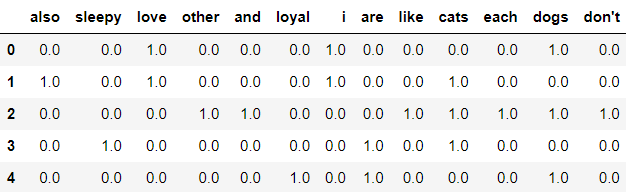### Implementation using CountVectorizer

The manual method despite being simple, has certain issues. Firstly, manually writing the code is time consuming and secondly, this method isn't scalable. Other than that, stop words are not removed which can lead to excess use of memory space. Keeping this in mind we shall work with the CountVectorizer module to create a bag of words for a proper dataset.

For this task, we shall be using the Customer Support Dataset.

Making the necessary imports -

``````import numpy as np
import pandas as pd

import re #used in data cleaning
import string
df = full_df[["text"]]
df["text"] = df["text"].astype(str)

``````

Considering that the dataset is quite large, we shall only be working with the first ten rows.

Output-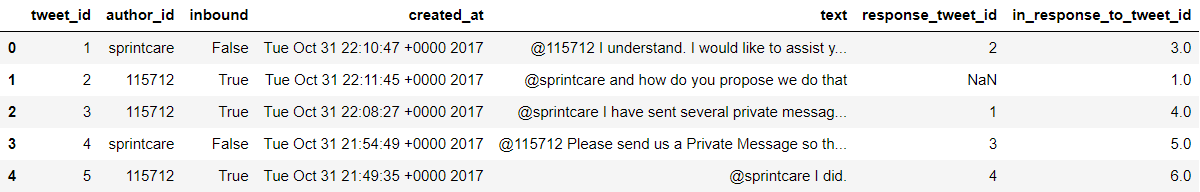Now we shall be cleaning the obtained data.

``````for x in list(df.columns):
if x not in ['tweet_id','text']:
del df[x]

ps = list(";?.:!,")

for p in ps:
df['text'] = df['text'].str.replace(p, '')

df['text'] = df['text'].str.replace("    ", " ")
df['text'] = df['text'].str.replace('"', '')
df['text'] = df['text'].apply(lambda x: x.replace('\t', ' '))
df['text'] = df['text'].str.replace("'s", "")
df['text'] = df['text'].apply(lambda x: x.replace('\n', ' '))
df['text'] = df['text'].apply(lambda x:x.lower())
df

def apply_regex_to_clean(txt):
txt = txt.lower()
txt = re.sub('[^a-zA-Z]', ' ', txt)
txt = re.sub('http\S+\s*', ' ', txt)
txt = re.sub('RT|cc', ' ', txt)
txt = re.sub('#\S+', '', txt)
txt = re.sub('@\S+', '  ', txt)
txt = re.sub('\s+', ' ', txt)
return txt
df['cleaned data'] = df['text'].apply(lambda x: apply_regex_to_clean(x))
del df['text']
df
``````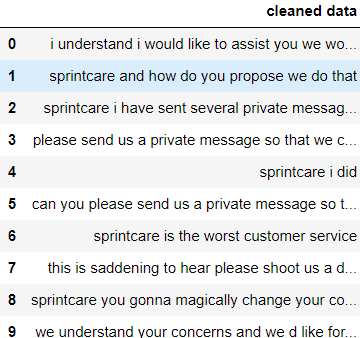Data is now cleaned and ready for use.

We shall be importing the Count Vectorizer functionality from sklearn's feature_extraction.text module. This shall convert the text to numerical data so that machines can work upon it easily.

``````from sklearn.feature_extraction.text import CountVectorizer
CountVec = CountVectorizer(ngram_range=(1,1),
stop_words=None)
#transform
Count_data = CountVec.fit_transform(df['cleaned data'])

#create dataframe
bag_of_words=pd.DataFrame(Count_data.toarray(),columns=CountVec.get_feature_names())
bag_of_words
``````

The Count Vectorizer initially takes two parameters - ngram_range and stop_ words. Ngrams refer to the number of words represented by each token. Here we take it's value to be (1,1) as we only want 1 word per token. The other parameter takes a list of stop words, which are removed from the corpus. We won't be removing any stop words for now, so we shall initialize it as 'None'.

Next, we shall use the fit_transform method to get our initial results-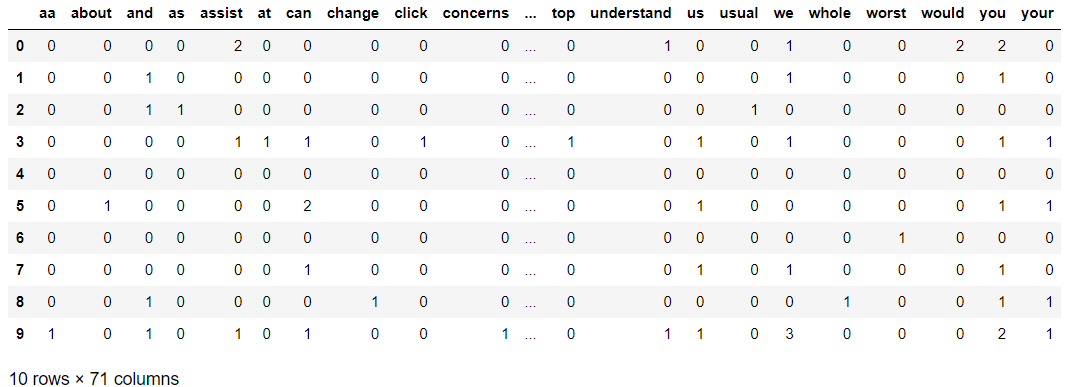Do note that the number of columns is quite large. This is to be expected, as the Bag Of Words approach is known to generate high dimensional data. To reduce the dimensionality somewhat, we can remove the stop words and regenerate the bag of words.

There are two approaches to Stopword removal. One is specifying the list of stopwords in the parameter, and the other is defining a function to do so. Since the first approach is clear already, we shall be using the second approach.

``````from nltk.corpus import stopwords
from nltk.tokenize import word_tokenize
def remove_stopwords(text):
stop_words = set(stopwords.words('english'))
tokens = word_tokenize(text)
filtered_tokens = [token for token in tokens if token.lower() not in stop_words]
filtered_text = ' '.join(filtered_tokens)
return filtered_text

df['filtered text'] = df['cleaned data'].apply(remove_stopwords)
del df['cleaned data']
df
``````

After removal of stopwords, the data looks something like this.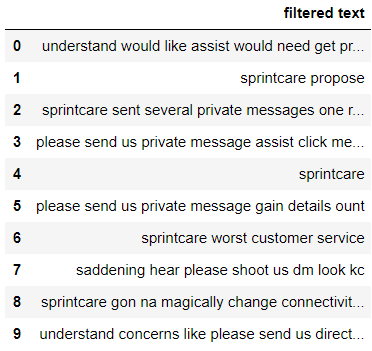Moving forward, we shall construct the Bag Of Words for this data.

``````Count_data = CountVec.fit_transform(df['filtered text'])

#create dataframe
Bag_Of_Words_NoSW=pd.DataFrame(Count_data.toarray(),columns=CountVec.get_feature_names())
Bag_Of_Words_NoSW
``````

Output -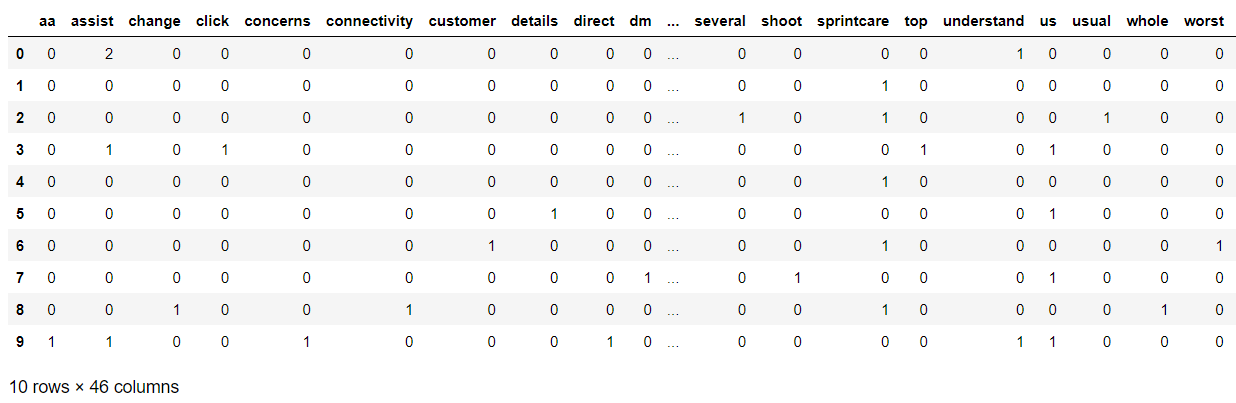As can be seen, the number of columns have been substantially reduced.

To sum up, Bag Of Words is a text representation technique wherein the underlying structure of the text is ignored and only the words are kept. This approach has both positive and negative sides, and hence any application of this technique should be after consideration of said sides.

## Comparison with other methods

Now that we have understood BOW, let's compare it with another popular method used in NLP- the tf-idf representation.

Bag-Of-WordsTF-IDF
BOW represents each word in the text document as a separate feature. TF-IDF represents text documents as a weighted sum of their constituent words, the weight depending upon the frequency and importance of said word.
BoW considers the frequency of words in a document. TF-IDF considers both the frequency of words in a document as well as their inverse frequency across the corpus, so as to to give more weight to words that are rare in the corpus.
BoW does not normalize the frequency of words. TF-IDF normalizes the frequency of words.
BoW is less robust to noisy data and stop words. TF-IDF is more robust to noisy data and stop words as it gives less weight to them.

On having a look at their characteristics, it can be said that both BoW and TF-IDF have their own strengths and weaknesses, and may perform better or worse than than the other depending upon the type of problem being solved. For example, BoW is better suited for document classification, sentiment analysis and other problems of similar nature, while TF-IDF is more efficient in tasks such as information retrieval and building recommendation systems.

## Question to consider

#### Q1) Which task is the BOW approach not suited for?

Sentiment Analysis.
Text Classification.
Sequence Modeling.
Information Retrieval.
Sequence Modeling is a task which BOW is not suited for, because the sequence of words and structure of the text matters here.

#### Q2) How can the number of words in the BOW be reduced?

Dimensionality Reduction techniques like PCA.
Removal of stopwords
Both A and B
Neither A nor B
Number of words in the BOW can be reduced by both PCA and stopword removal, as the BOW is implemented inn the form of a dataframe.

With this article at OpenGenus, you must have the complete idea of Bag of Words (BoW) in NLP.#### Ambarish Deb

Final Year Computer Science Student at Sri Guru Gobind Singh College Of Commerce; ML Intern at OpenGenus.